Next: 2.2.1 Reactor-Scale Transport Up: 2. Process Modeling Previous: 2.1 Continuum Approach

# 2.2 Transport Kinetics

In dry processes, where the wafer is exposed to a gas phase, the flux distribution of particles on the surface is usually the crucial factor for the local deposition or etching rates. Common models for the particle transport within the process chamber to the wafer surface divide the gas phase region by a plane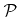just above the surfaceas depicted in Figure 2.1 [32,80]. A first model describes the transport within the reactor-scale region toand allows the determination of the flux distribution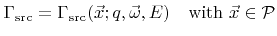(2.2)

which describes the number of arriving particles of species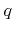with incident direction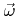and energy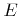per unit area. Hereis a unit vector. The total flux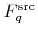of particles of typecrossingis the integral over all directions and energies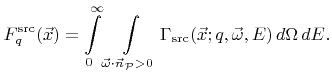(2.3)

Here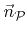denotes the normal vector topointing towards(see Figure 2.1).

A second model describes the continued particle transport fromtowithin the feature-scale region using (2.2) as a boundary condition. The advantage of dividing the gas phase region is that the particle transport acts on different length scales, and hence, different transport models can be applied to each region.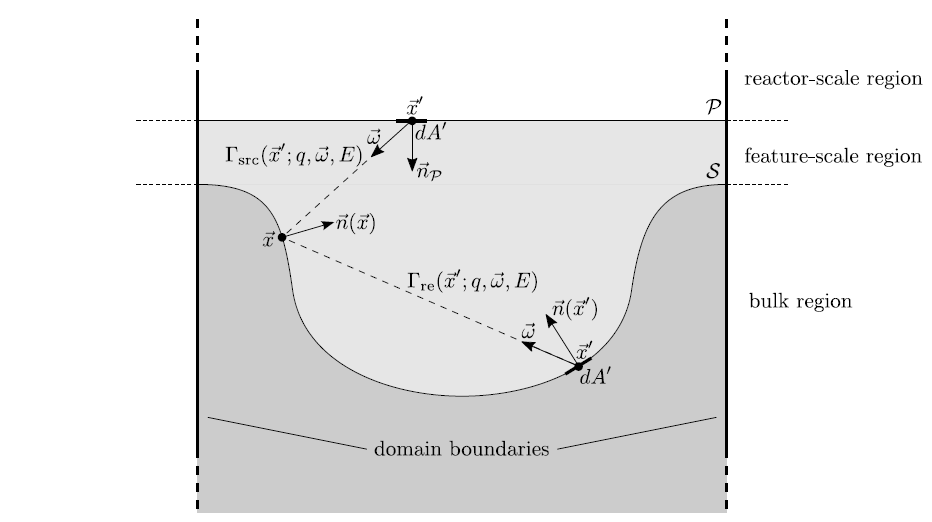SubsectionsNext: 2.2.1 Reactor-Scale Transport Up: 2. Process Modeling Previous: 2.1 Continuum Approach

Otmar Ertl: Numerical Methods for Topography Simulation